Function model_performance() calculates various performance measures for classification and regression models. For classification models following measures are calculated: F1, accuracy, recall, precision and AUC. For regression models following measures are calculated: mean squared error, R squared, median absolute deviation.

model_performance(explainer, ..., cutoff = 0.5)

## Arguments

explainer

a model to be explained, preprocessed by the explain function

...

other parameters

cutoff

a cutoff for classification models, needed for measures like recall, precision, ACC, F1. By default 0.5.

## Value

An object of the class model_performance.

It's a list with following fields:

• residuals - data frame that contains residuals for each observation

• measures - list with calculated measures that are dedicated for the task, whether it is regression, binary classification or multiclass classification.

• type - character that specifies type of the task.

## Examples

# \donttest{
# regression

library("ranger")
apartments_ranger_model <- ranger(m2.price~., data = apartments, num.trees = 50)
explainer_ranger_apartments  <- explain(apartments_ranger_model, data = apartments[,-1],
y = apartments$m2.price, label = "Ranger Apartments") #> Preparation of a new explainer is initiated #> -> model label : Ranger Apartments #> -> data : 1000 rows 5 cols #> -> target variable : 1000 values #> -> predict function : yhat.ranger will be used ( default ) #> -> predicted values : No value for predict function target column. ( default ) #> -> model_info : package ranger , ver. 0.14.1 , task regression ( default ) #> -> predicted values : numerical, min = 1869.304 , mean = 3488.634 , max = 6164.522 #> -> residual function : difference between y and yhat ( default ) #> -> residuals : numerical, min = -415.6353 , mean = -1.614668 , max = 649.3253 #> A new explainer has been created! model_performance_ranger_aps <- model_performance(explainer_ranger_apartments ) model_performance_ranger_aps #> Measures for: regression #> mse : 24901.87 #> rmse : 157.8033 #> r2 : 0.9696787 #> mad : 89.72723 #> #> Residuals: #> 0% 10% 20% 30% 40% 50% #> -415.635333 -166.039673 -115.898733 -81.885100 -56.288733 -28.946381 #> 60% 70% 80% 90% 100% #> 6.227667 46.894300 104.919600 206.875317 649.325333 plot(model_performance_ranger_aps)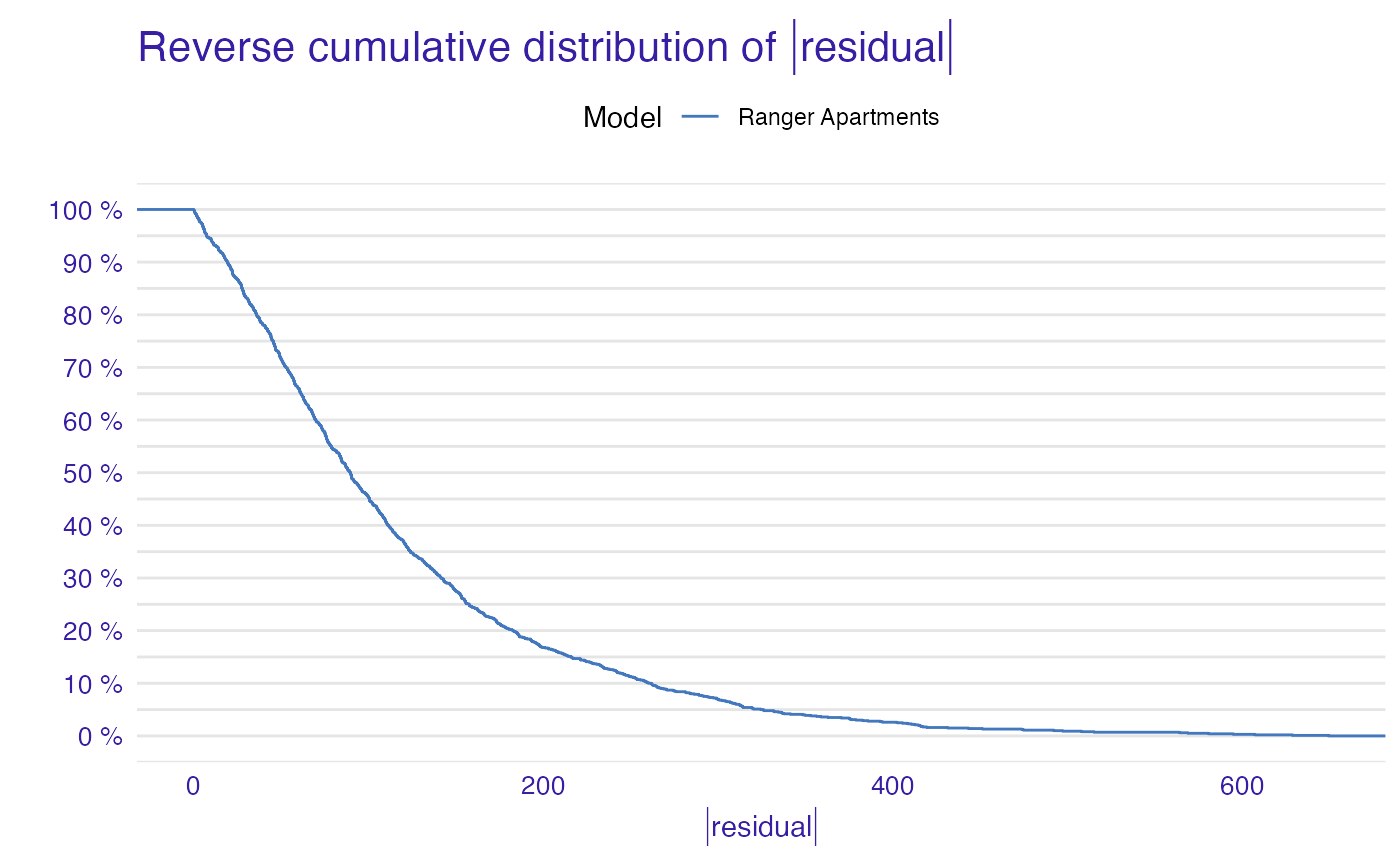plot(model_performance_ranger_aps, geom = "boxplot")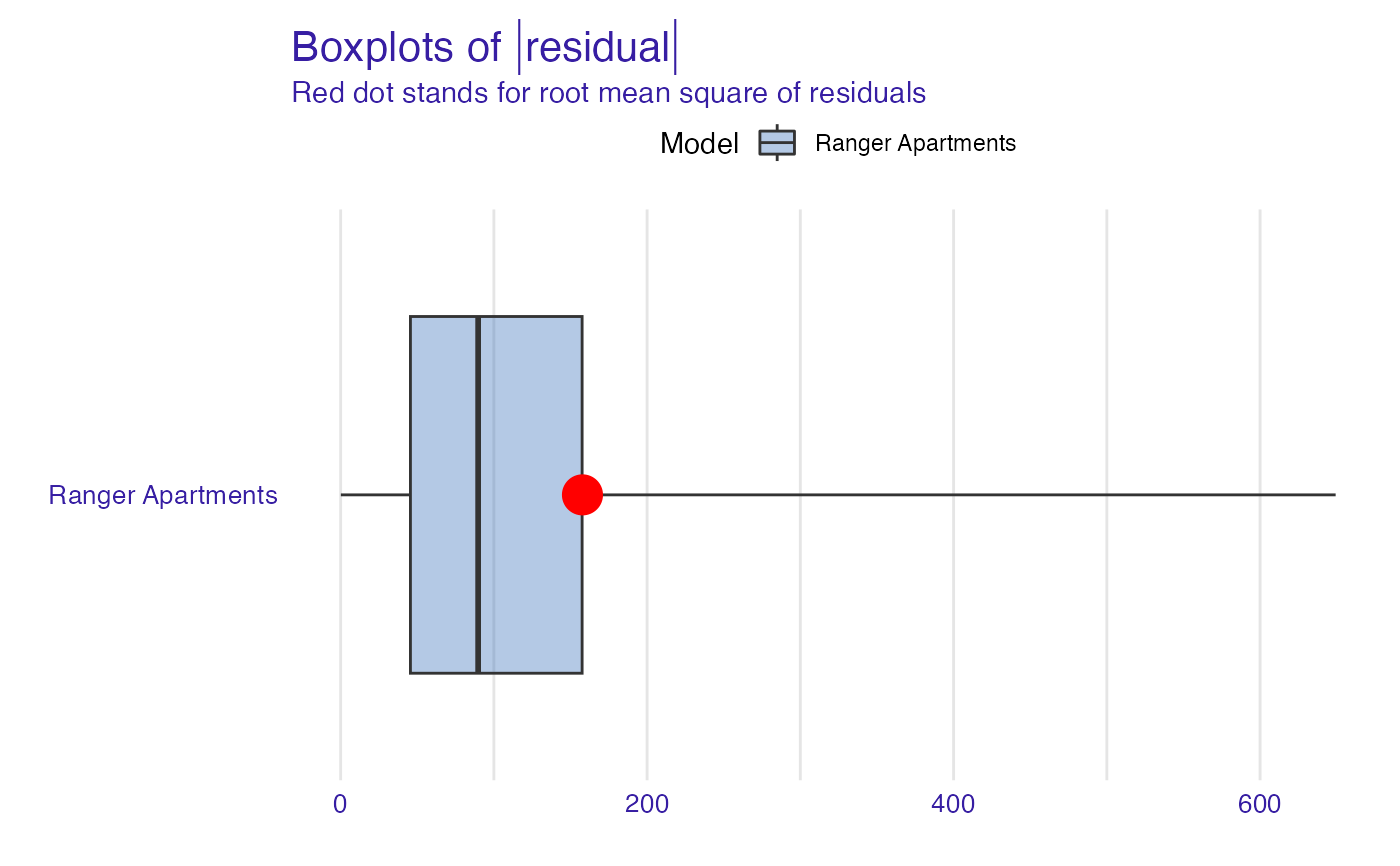plot(model_performance_ranger_aps, geom = "histogram")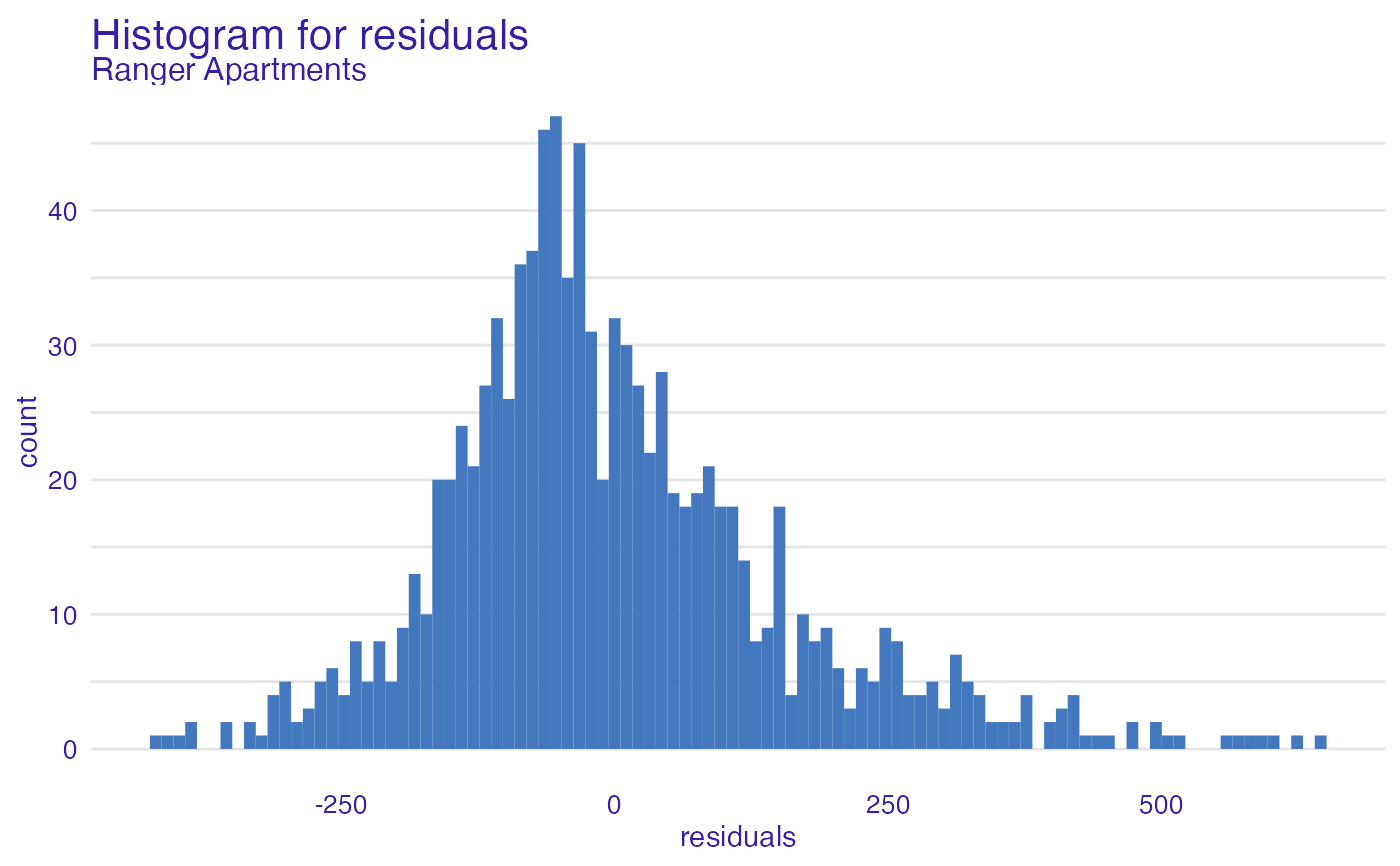# binary classification titanic_glm_model <- glm(survived~., data = titanic_imputed, family = "binomial") explainer_glm_titanic <- explain(titanic_glm_model, data = titanic_imputed[,-8], y = titanic_imputed$survived)
#> Preparation of a new explainer is initiated
#>   -> model label       :  lm  (  default  )
#>   -> data              :  2207  rows  7  cols
#>   -> target variable   :  2207  values
#>   -> predict function  :  yhat.glm  will be used (  default  )
#>   -> predicted values  :  No value for predict function target column. (  default  )
#>   -> model_info        :  package stats , ver. 4.2.3 , task classification (  default  )
#>   -> predicted values  :  numerical, min =  0.008128381 , mean =  0.3221568 , max =  0.9731431
#>   -> residual function :  difference between y and yhat (  default  )
#>   -> residuals         :  numerical, min =  -0.9628583 , mean =  -2.569729e-10 , max =  0.9663346
#>   A new explainer has been created!
model_performance_glm_titanic <- model_performance(explainer_glm_titanic)
model_performance_glm_titanic
#> Measures for:  classification
#> recall     : 0.5738397
#> precision  : 0.7472527
#> f1         : 0.6491647
#> accuracy   : 0.8001812
#> auc        : 0.8115462
#>
#> Residuals:
#>          0%         10%         20%         30%         40%         50%
#> -0.96285832 -0.32240247 -0.23986439 -0.19544185 -0.14842925 -0.11460334
#>         60%         70%         80%         90%        100%
#> -0.06940964  0.06185475  0.29607060  0.72120412  0.96633458
plot(model_performance_glm_titanic)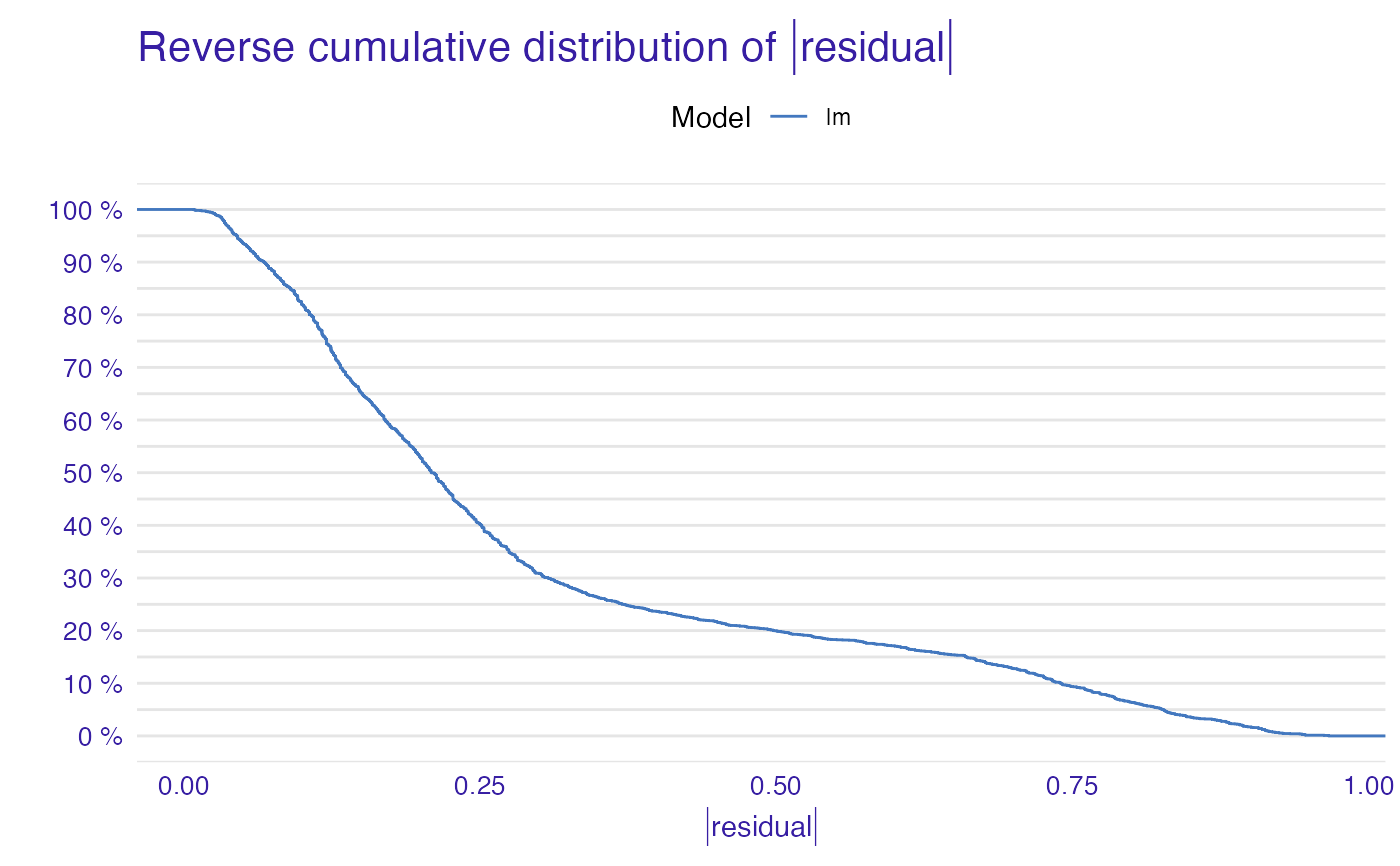plot(model_performance_glm_titanic, geom = "boxplot")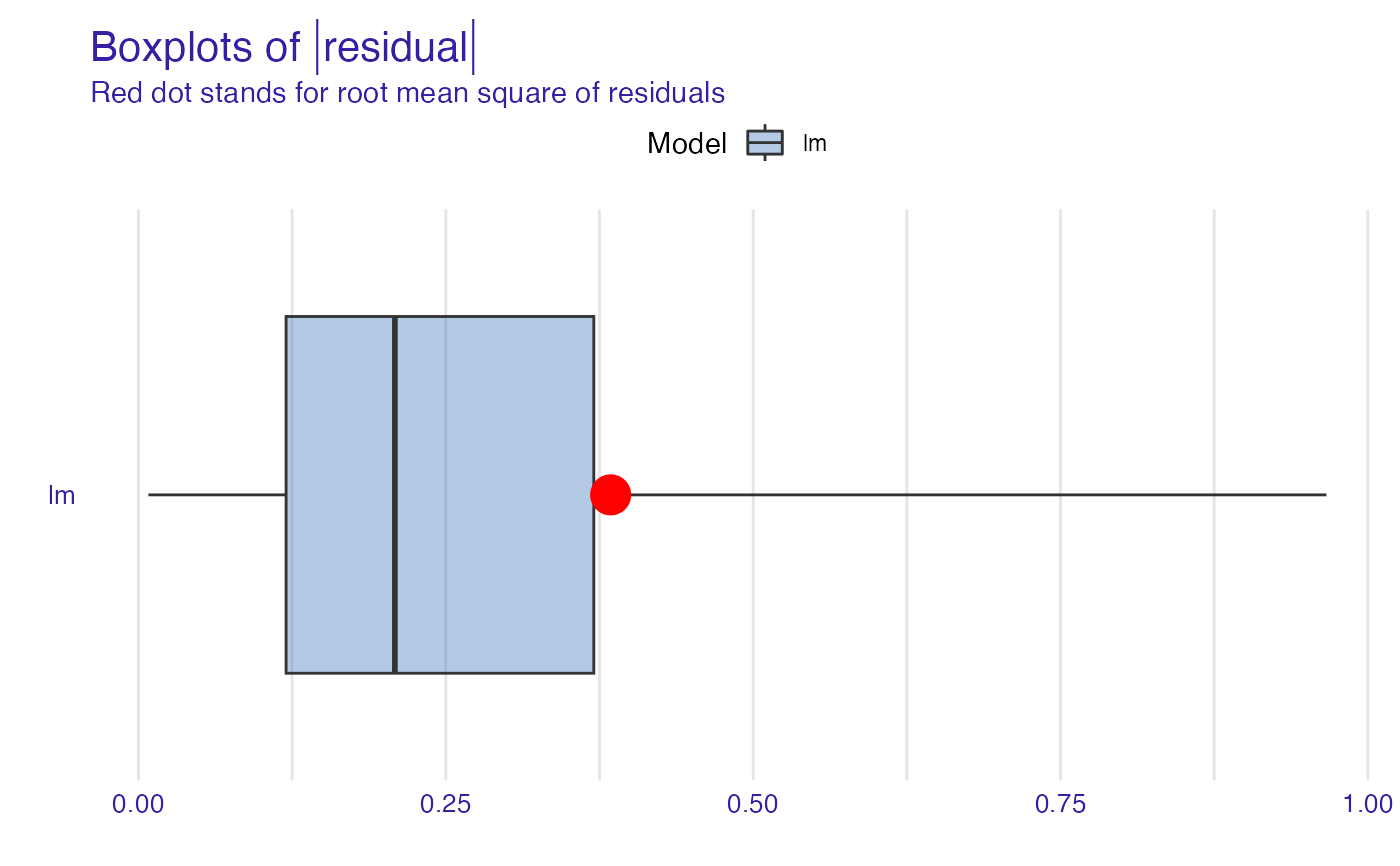plot(model_performance_glm_titanic, geom = "histogram")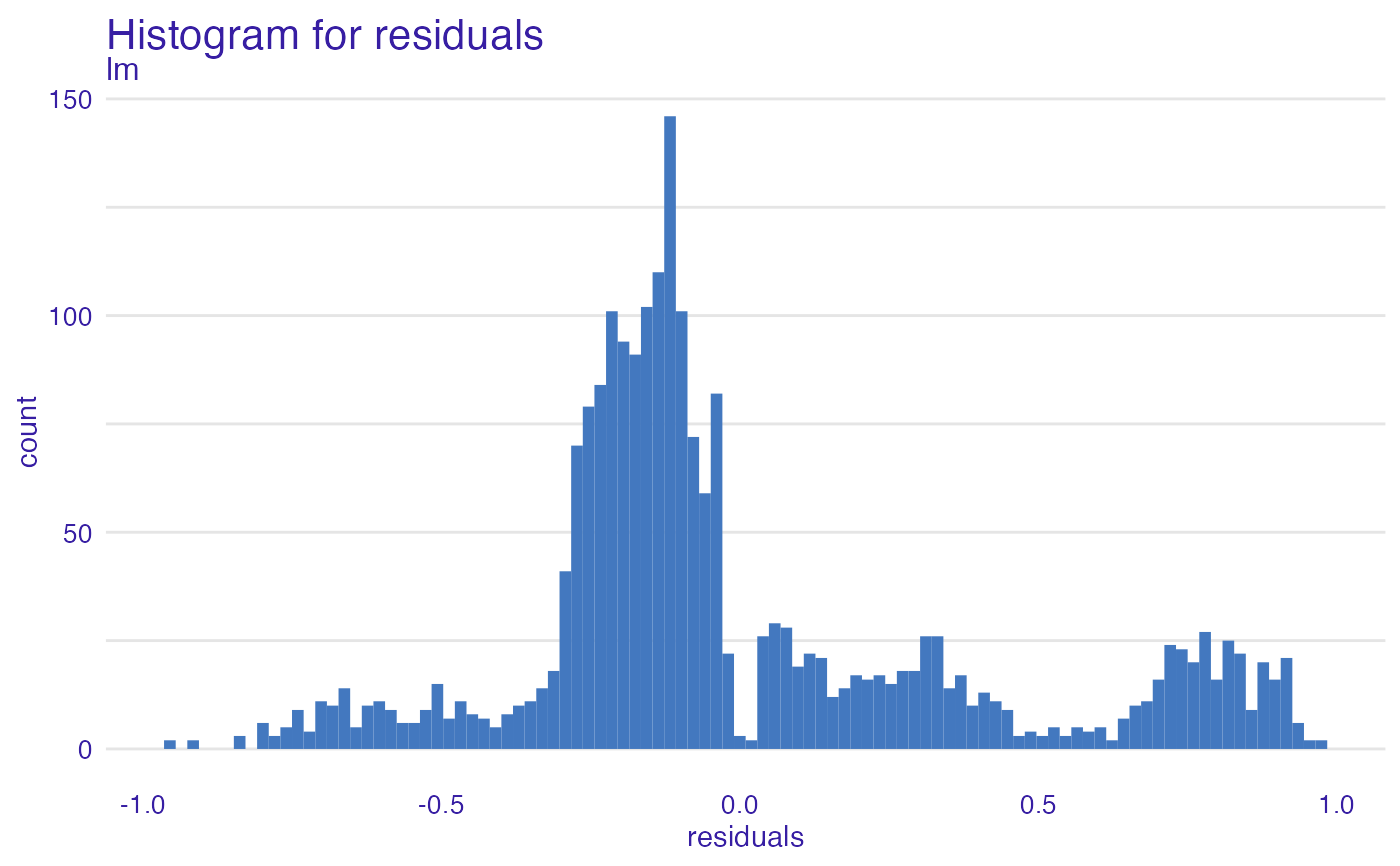# multilabel classification

HR_ranger_model <- ranger(status~., data = HR, num.trees = 50,
probability = TRUE)
explainer_ranger_HR  <- explain(HR_ranger_model, data = HR[,-6],
y = HR\$status, label = "Ranger HR")
#> Preparation of a new explainer is initiated
#>   -> model label       :  Ranger HR
#>   -> data              :  7847  rows  5  cols
#>   -> target variable   :  7847  values
#>   -> predict function  :  yhat.ranger  will be used (  default  )
#>   -> predicted values  :  No value for predict function target column. (  default  )
#>   -> model_info        :  package ranger , ver. 0.14.1 , task multiclass (  default  )
#>   -> predicted values  :  predict function returns multiple columns:  3  (  default  )
#>   -> residual function :  difference between 1 and probability of true class (  default  )
#>   -> residuals         :  numerical, min =  0 , mean =  0.2774276 , max =  0.8822464
#>   A new explainer has been created!
model_performance_ranger_HR <- model_performance(explainer_ranger_HR)
model_performance_ranger_HR
#> Measures for:  multiclass
#> micro_F1   : 0.8682299
#> macro_F1   : 0.8665459
#> w_macro_F1 : 0.8672412
#> accuracy   : 0.8682299
#> w_macro_auc: 0.9767981
#> cross_entro: 2976.313
#>
#> Residuals:
#>         0%        10%        20%        30%        40%        50%        60%
#> 0.00000000 0.02883482 0.06314101 0.11658845 0.17280386 0.23823724 0.31082295
#>        70%        80%        90%       100%
#> 0.39140746 0.48752605 0.59540191 0.88224638
plot(model_performance_ranger_HR)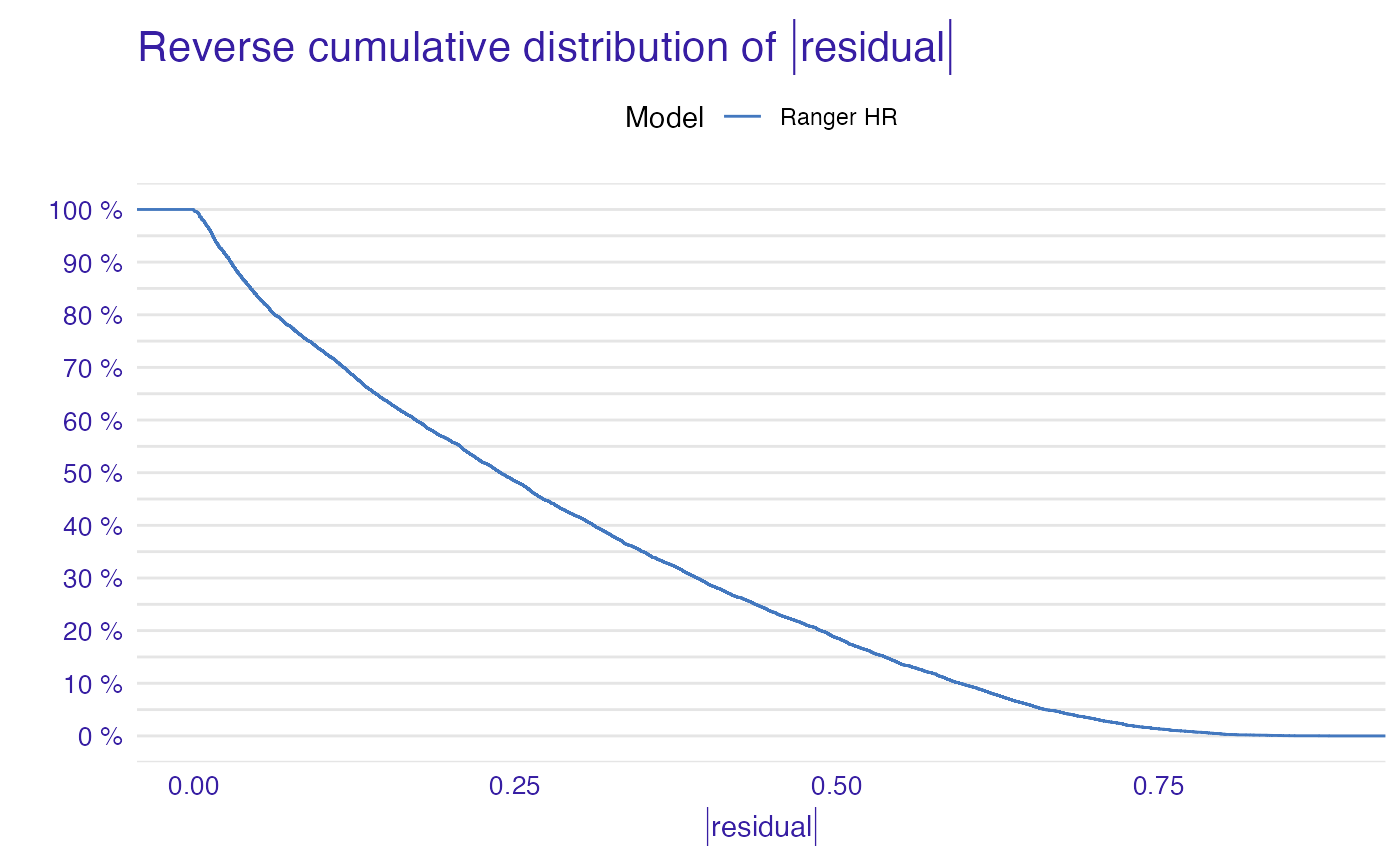plot(model_performance_ranger_HR, geom = "boxplot")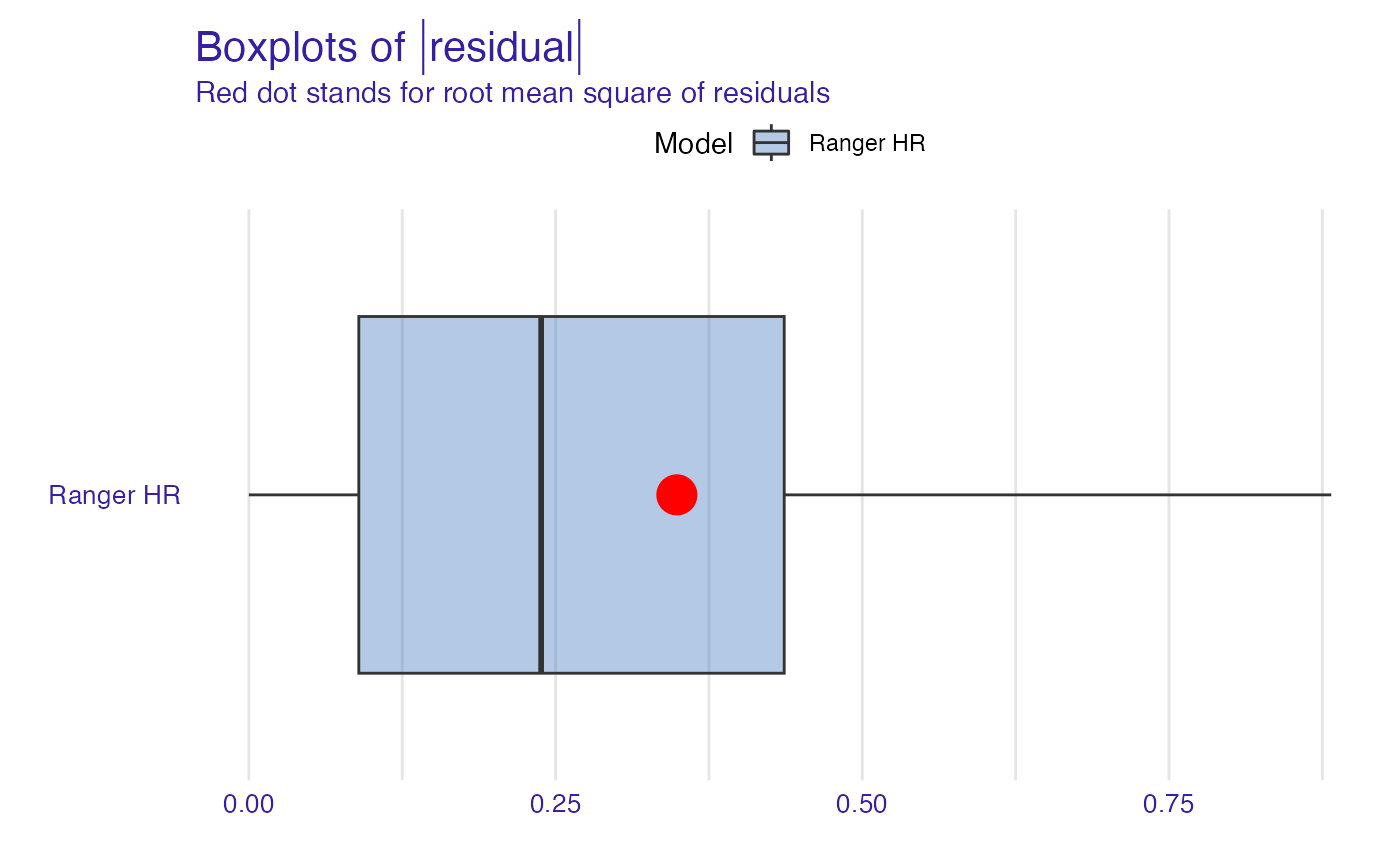plot(model_performance_ranger_HR, geom = "histogram")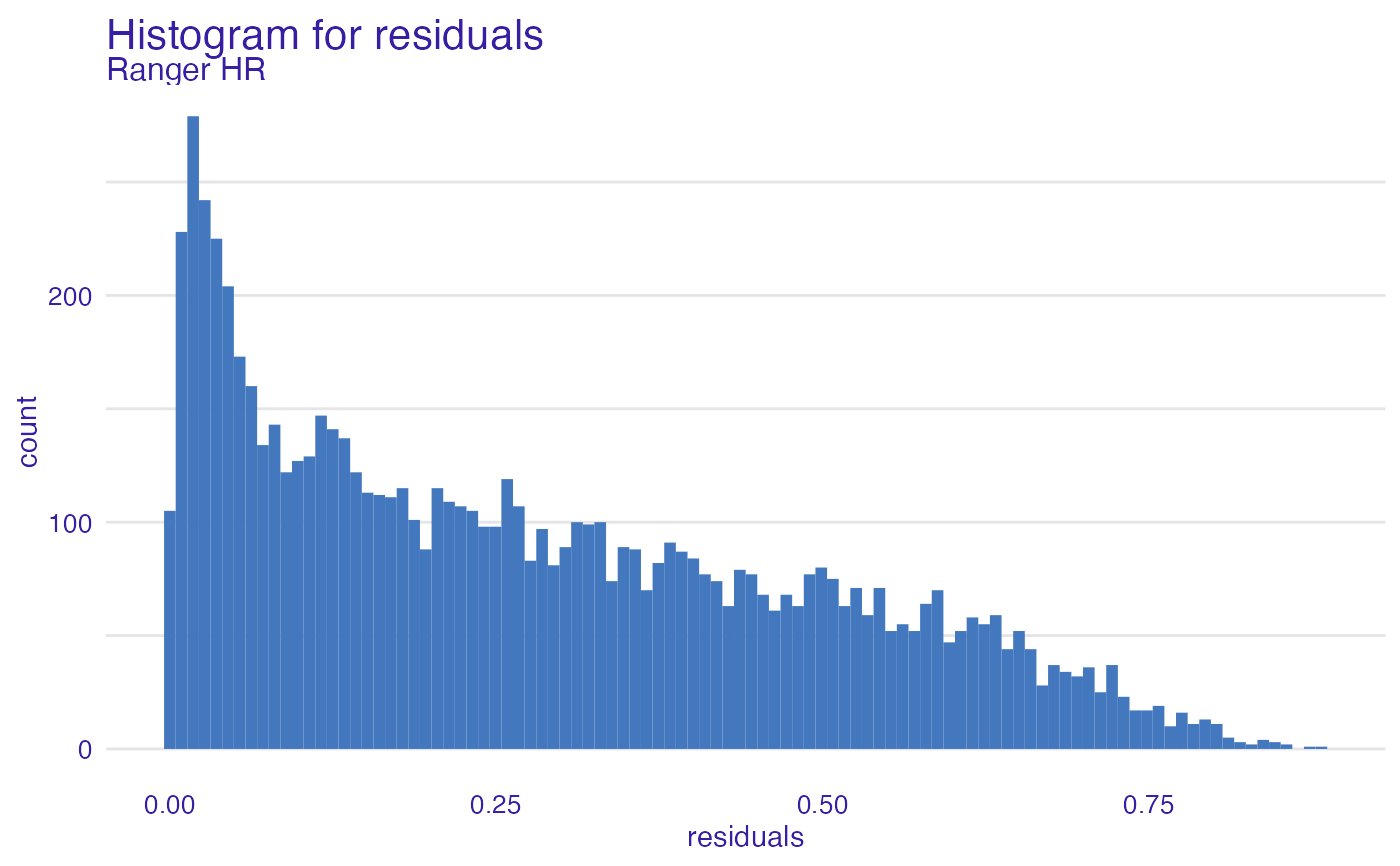# }